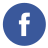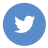### Java Methods

java

In Java, a method is a block of code that performs a specific task. A method can be called from other parts of the program to execute the task it contains.

Here is the syntax for declaring a method in Java:

``````accessModifier returnType methodName(parameterList) {
// method body
}``````

Here is an example of a method in Java:

``````public int add(int x, int y) {
int sum = x + y;
return sum;
}``````

In this example, add is a method that takes two integers as parameters and returns their sum. The return statement is used to return a value from the method.

To call a method, use its name followed by a list of arguments in parentheses:

``````int a = 3;
int b = 4;
int c = add(a, b); // c is 7``````

A method can also have no return value and no parameters. In this case, the returnType is void and the parameterList is empty.

``````public void printHello() {
System.out.println("Hello!");
}``````

To call this method, simply use its name with no arguments:

``printHello(); // prints "Hello!"``